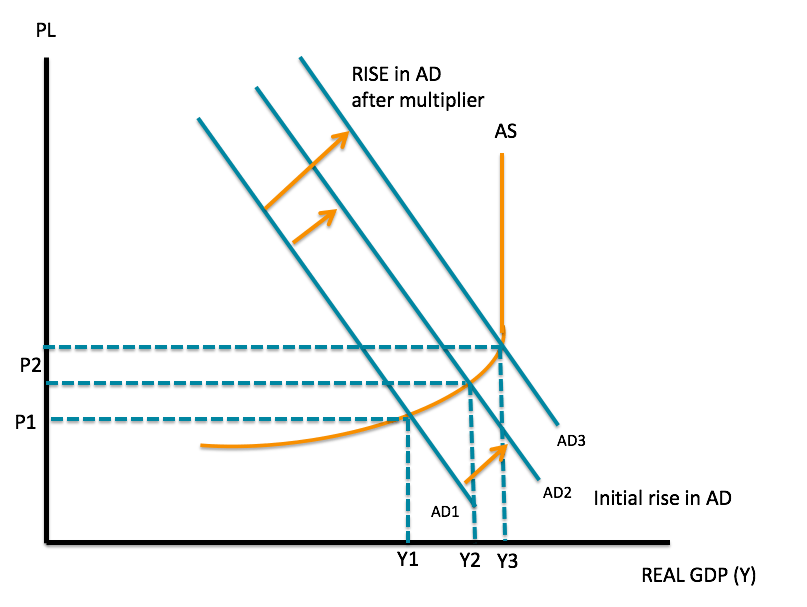# Keynesian Multiplier

An increase in private consumption or investment expenditure, or net government spending raises the total GDP by more than the amount of the increase

## What is the Keynesian Multiplier?

The Keynesian Multiplier states that an increase in private consumption expenditure, investment expenditure, or net government spending (gross government spending – government tax revenue) raises the total Gross Domestic Product (GDP) by more than the amount of the increase. Therefore, if private consumption expenditure increases by 10 units, the total GDP will increase by more than 10 units.

### Keynesian Economic Theory

In 1936, economist John Maynard Keynes published a text that would change the course of economic thought. Titled “The General Theory of Employment, Interest, and Money,” or simply as “The General Theory,” it is considered one of the classical works in economics. The book attempted to explain short-term economic fluctuations in general, especially the fluctuations observed during the Great Depression in the early 1930s.

The main idea put forth by Keynes in The General Theory was that recessions and depressions could occur because of inadequate demand in the market for goods and services.

The General Theory was intended not just for economists but also for policymakers across the world. In response to widespread unemployment and low levels of economic activity across the world, Keynes called for an increase in government spending in order to boost demand for goods and services in the market. The thinking went against the existing classical economic policy of laissez-faire and minimal government interference.

### Components of the Keynesian Theory

The three main components of the Keynesian Theory are:

1. Aggregate demand is influenced by the decisions in the private and public sector. The level of demand by the private sector could exert an effect on macroeconomic conditions. For example, a decrease in aggregate spending can bring the economy into a recession. However, the negative impact of private decision-making can be mitigated through government intervention with a fiscal or monetary stimulus.
2. Price such as wages are often slow to respond to changes in demand and supply. It is why there are many instances of a shortage or an excess in the supply of labor.
3. A change in aggregate demand causes the greatest impact on the output and employment in the economy. Keynesian economic theory says that spending by consumers and the government, investment, and exports will increase the level of output. Even a change in one the components will cause total output to change.

The concept of the change in aggregate demand was used to develop the Keynesian multiplier. It says that the output in the economy is a multiple of the increase or decrease in spending. If the fiscal multiplier is greater than 1, then a \$1 increase in spending will increase the total output by a value greater than \$1.(Image) The increase from AD1 to AD2 leads to an increase in output from Y1 to Y2. But with a multiplier, there is a rise to AD and a further increase in output at Y3.

### Calculating the Keynesian Multiplier

The value of the multiplier depends on the marginal propensity to consume and the marginal propensity to save.

#### 1. Marginal Propensity to Save

The change in total savings as a result of a change in total income is known as the marginal propensity to save. When an individual’s income increases, the marginal propensity to save (MPS) measures the proportion of income the person saves rather than spend on goods and services. It is calculated as MPS = ΔS / ΔY.

Suppose an individual receives a year-end bonus of \$600 and spends \$300 on goods and services. The MPS is (600 – 300) / 600 = 0.5.

#### 2. Marginal Propensity to Consume

The change in total consumption as a result of a change in total income is known as the marginal propensity to consume. The marginal propensity to consume (MPC) measures how consumer spending changes with a change in income. Using the figures above, the MPC is ΔC / ΔY = 300/600 = 0.5.

The Keynesian Theory states that an increase in production leads to an increase in the level of income and therefore, an increase in spending. The value of MPC allows us to calculate the size of the multiplier using the formula:

1 / (1 – MPC) = 1 / (1 – 0.5) = 2

It means that every \$1 of new income will generate \$2 of extra income.